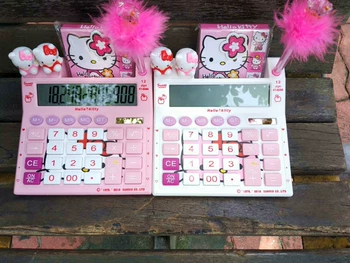#S o u t h K o r e a ' s c r e a t i v e c a r t o o n H o l l y k i t t y c o m p u t e r s o l a r p o r t a b l e c o m p u t e r p e n p u t b u s i n e s s c a r d c o m p u t e r

Updated Wednesday 13-Nov-2019## S o u t h K o r e a ' s c r e a t i v e c a r t o o n H o l l y k i t t y c o m p u t e r s o l a r p o r t a b l e c o m p u t e r p e n p u t b u s i n e s s c a r d c o m p u t e r 0

THB 154.75